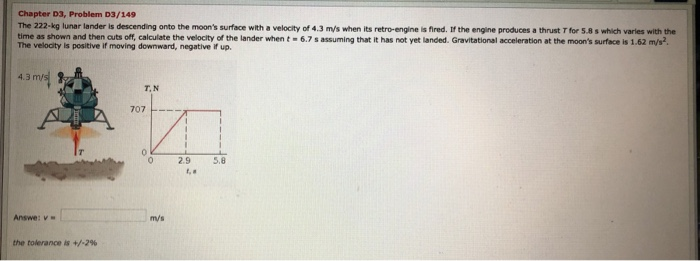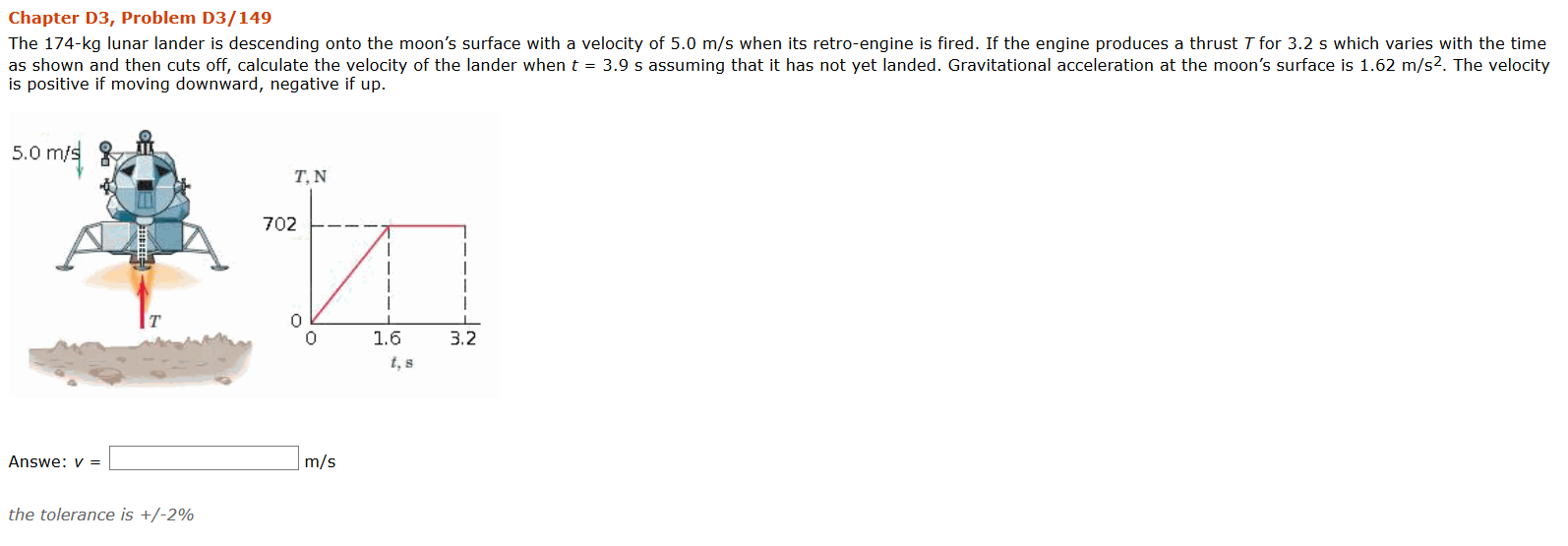Question

# The Apollo Lunar Module was used to make the transition from the spacecraft to the moon's surface and back.

The Apollo Lunar Module was used to make the transition from the spacecraft to the moon's surface and back. Consider a similar module for landing on the surface ofMars. Use conservation of mechanical energy to answer these questions.

(a) As the lander is descending, if the pilot decides to shut down the engine when the lander is at a height of 1.8 m, (this may not be a safe height to shut down theengine) and the velocity of the lander (relative to the surface of the planet) is 1.2 m/s what will be velocity of the lander at impact? Note: g on the surface of marsis about 0.4 times that on the surface of the Earth.

(b) In the case of the lunar module an impact velocity of 3.0 m/s or less was essential for a safe landing. Assuming this to be the case for the Mars lander as well,at what maximum height could the pilot shut down the engines to ensure a safe landing. Assume the velocity v0 at the time the engine is shut down is 1.2 m/s.

a) use X=Xo+Vot+.5at^2 to find t
then use V=Vo+at^2 to find v :D

b) same concept
A. the kinematic equation you must use is:
v^2 = u^2 +2as
now for the question we have u= 1.2 s= 1.8 and g= 9.8/4 = 2.45
now v^2 = 1.2^2 + 2*2.45*1.8 => v= root(10.26) =3.2m/s

B.Again we the same formula only we need to find s in the equation
we know that V=3m/s u =1.2m/s a= 2.45m/s^2

3^2 = 1.2^2 +2*2.45*s
=> s= (9- 1.44)/4.9 = 1.54 m

#### Earn Coins

Coins can be redeemed for fabulous gifts.

Similar Homework Help Questions
• ### Physics question regarding mechanical energy

The Apollo Lunar Module was used to make the transition from the spacecraft to the moon's surface and back. Consider a similar module for landing on the surface ofMars. Use conservation of mechanical energy to answer these questions.(a) As the lander is descending, if the pilot decides to shut down the engine when the lander is at a height of 1.8 m, (this may not be a safe height to shut down theengine) and the velocity of the lander (relative...

• ### On Apollo Moon missions, the lunar module would blast off from the Moon's surface and dock...

On Apollo Moon missions, the lunar module would blast off from the Moon's surface and dock with the command module in lunar orbit. After docking, the lunar module would be jettisoned and allowed to crash back onto the lunar surface. Seismometers placed on the Moon's surface by the astronauts would then pick up the resulting seismic waves. Find the impact speed of the lunar module, given that it is jettisoned from an orbit 130 km above the lunar surface moving...

• ### A lunar lander is descending toward the moon's surface. Until the lander reaches the surface, its...

A lunar lander is descending toward the moon's surface. Until the lander reaches the surface, its height above the surface of the moon is given by y(t)=b−ct+dt^2 , where b = 710m is the initial height of the lander above the surface, c = 64.0m/s, and d = 1.03 m/s^2. (A) What is the initial velocity of the lander, at t = 0? (B) What is the velocity of the lander just before it reaches the lunar surface?

• ### Chapter D3, Problem 03/149 The 222-kg lunar lander is descending onto the moon's surface with a...Chapter D3, Problem 03/149 The 222-kg lunar lander is descending onto the moon's surface with a velocity of 4.3 m/s when its retro-engine is fired. If the engine produces a thrust T for 5.8 s which varies with the time as shown and then cuts off, calculate the velocity of the lander when t - 6.7 s assuming that it has not yet landed. Gravitational acceleration at the moon's surface is 1.62 m/s? The velocity is positive if moving downward,...

• ### Chapter D3, Problem D3/149 The 174-kg lunar lander is descending onto the moon's surface with a...Chapter D3, Problem D3/149 The 174-kg lunar lander is descending onto the moon's surface with a velocity of 5.0 m/s when its retro-engine is fired. If the engine produces a thrust T for 3.2 s which varies with the time as shown and then cuts off, calculate the velocity of the lander when t = 3.9 s assuming that it has not yet landed. Gravitational acceleration at the moon's surface is 1.62 m/s2. The velocity is positive if moving downward,...

• ### Where is the Kuiper Belt located? Question 1 options: Between Earth and Mars Between Jupiter and...Where is the Kuiper Belt located? Question 1 options: Between Earth and Mars Between Jupiter and Saturn Between Uranus and Neptune Beyond Neptune Save Question 2 (0.5 points) What is the name of the first man made satellite launched in space? Question 2 options: Explorer 1 Sputnik 1 Soyuz Salyut 1 Save Question 3 (0.5 points) What is the name of the first privately owned spacecraft to dock with the International Space Station? Question 3 options: CST-100 DreamChaser Falcon 9...